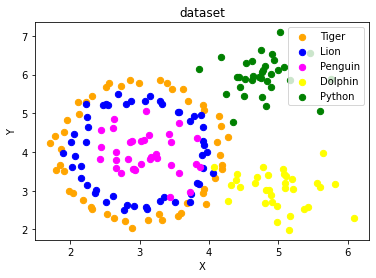## Data Generation

### Generate Synthetical Data with PythonA problem with machine learning, especially when you are starting out and want to learn about the algorithms, is that it is often difficult to get suitable test data. Some cost a lot of money, others are not freely available because they are protected by copyright. Therefore, artificially generated test data can be a solution in some cases.

For this reason, this chapter of our tutorial deals with the artificial generation of data. This chapter is about creating artificial data. In the previous chapters of our tutorial we learned that Scikit-Learn (sklearn) contains different data sets. On the one hand, there are small toy data sets, but it also offers larger data sets that are often used in the machine learning community to test algorithms or also serve as a benchmark. It provides us with data coming from the 'real world'.

All this is great, but in many cases this is still not sufficient. Maybe you find the right kind of data, but you need more data of this kind or the data is not completely the kind of data you were looking for, e.g. maybe you need more complex or less complex data. This is the point where you should consider to create the data yourself. Here, sklearn offers help. It includes various random sample generators that can be used to create custom-made artificial datasets. Datasets that meet your ideas of size and complexity.

The following Python code is a simple example in which we create artificial weather data for some German cities. We use Pandas and Numpy to create the data:

import numpy as np
import pandas as pd

cities = ['Berlin', 'Frankfurt', 'Hamburg',
'Nuremberg', 'Munich', 'Stuttgart',
'Hanover', 'Saarbruecken', 'Cologne',
'Constance', 'Freiburg', 'Karlsruhe'
]

n= len(cities)
data = {'Temperature': np.random.normal(24, 3, n),
'Humidity': np.random.normal(78, 2.5, n),
'Wind': np.random.normal(15, 4, n)
}
df = pd.DataFrame(data=data, index=cities)
df

Output:
Temperature Humidity Wind
Berlin 20.447301 75.516079 12.566956
Frankfurt 27.319526 77.010523 11.800371
Hamburg 24.783113 80.200985 14.489432
Nuremberg 25.823295 76.430166 19.903070
Munich 21.037610 81.589453 17.677132
Stuttgart 25.560423 75.384543 20.832011
Hanover 22.073368 81.704236 12.421998
Saarbruecken 25.722280 80.131432 10.694502
Cologne 25.658240 79.430957 16.360829
Constance 29.221204 75.626223 17.281035
Freiburg 25.625042 81.227281 6.850105
Karlsruhe 26.245587 81.546979 11.787846

### Another Example

We will create artificial data for four nonexistent types of flowers:

• Flos Pythonem
• Flos Java
• Flos Margarita
• Flos artificialis

The RGB avarage colors values are correspondingly:

• (255, 0, 0)
• (245, 107, 0)
• (206, 99, 1)
• (255, 254, 101)

The average diameter of the calyx is:

• 3.8
• 3.3
• 4.1
• 2.9
Flos pythonem
(254, 0, 0)
Flos Java
(245, 107, 0)
Flos margarita
(206, 99, 1)
Flos artificialis
(255, 254, 101)
import matplotlib.pyplot as plt
import numpy as np
import pandas as pd

from scipy.stats import truncnorm

def truncated_normal(mean=0, sd=1, low=0, upp=10, type=int):
return truncnorm(
(low - mean) / sd, (upp - mean) / sd, loc=mean, scale=sd)

def truncated_normal_floats(mean=0, sd=1, low=0, upp=10, num=100):
res = truncated_normal(mean=mean, sd=sd, low=low, upp=upp)
return res.rvs(num)

def truncated_normal_ints(mean=0, sd=1, low=0, upp=10, num=100):
res = truncated_normal(mean=mean, sd=sd, low=low, upp=upp)
return res.rvs(num).astype(np.uint8)

# number of items for each flower class:
number_of_items_per_class = [190, 205, 230, 170]
flowers = {}
# flos Pythonem:
number_of_items = number_of_items_per_class
reds = truncated_normal_ints(mean=254, sd=18, low=235, upp=256,
num=number_of_items)
greens = truncated_normal_ints(mean=107, sd=11, low=88, upp=127,
num=number_of_items)
blues = truncated_normal_ints(mean=0, sd=15, low=0, upp=20,
num=number_of_items)
calyx_dia = truncated_normal_floats(3.8, 0.3, 3.4, 4.2,
num=number_of_items)
data = np.column_stack((reds, greens, blues, calyx_dia))
flowers["flos_pythonem"] = data

# flos Java:
number_of_items = number_of_items_per_class
reds = truncated_normal_ints(mean=245, sd=17, low=226, upp=256,
num=number_of_items)
greens = truncated_normal_ints(mean=107, sd=11, low=88, upp=127,
num=number_of_items)
blues = truncated_normal_ints(mean=0, sd=10, low=0, upp=20,
num=number_of_items)
calyx_dia = truncated_normal_floats(3.3, 0.3, 3.0, 3.5,
num=number_of_items)
data = np.column_stack((reds, greens, blues, calyx_dia))
flowers["flos_java"] = data

# flos Java:
number_of_items = number_of_items_per_class
reds = truncated_normal_ints(mean=206, sd=17, low=175, upp=238,
num=number_of_items)
greens = truncated_normal_ints(mean=99, sd=14, low=80, upp=120,
num=number_of_items)
blues = truncated_normal_ints(mean=1, sd=5, low=0, upp=12,
num=number_of_items)
calyx_dia = truncated_normal_floats(4.1, 0.3, 3.8, 4.4,
num=number_of_items)
data = np.column_stack((reds, greens, blues, calyx_dia))
flowers["flos_margarita"] = data

# flos artificialis:
number_of_items = number_of_items_per_class
reds = truncated_normal_ints(mean=255, sd=8, low=2245, upp=2255,
num=number_of_items)
greens = truncated_normal_ints(mean=254, sd=10, low=240, upp=255,
num=number_of_items)
blues = truncated_normal_ints(mean=101, sd=5, low=90, upp=112,
num=number_of_items)
calyx_dia = truncated_normal_floats(2.9, 0.4, 2.4, 3.5,
num=number_of_items)
data = np.column_stack((reds, greens, blues, calyx_dia))
flowers["flos_artificialis"] = data

data = np.concatenate((flowers["flos_pythonem"],
flowers["flos_java"],
flowers["flos_margarita"],
flowers["flos_artificialis"]
), axis=0)

# assigning the labels
target = np.zeros(sum(number_of_items_per_class)) # 4 flowers
previous_end = 0
for i in range(1, 5):
num = number_of_items_per_class[i-1]
beg = previous_end
target[beg: beg + num] += i
previous_end = beg + num

conc_data = np.concatenate((data, target.reshape(target.shape, 1)),
axis=1)

np.savetxt("data/strange_flowers.txt", conc_data, fmt="%2.2f",)

import matplotlib.pyplot as plt

target_names = list(flowers.keys())
feature_names = ['red', 'green', 'blue', 'calyx']
n = 4
fig, ax = plt.subplots(n, n, figsize=(16, 16))

colors = ['blue', 'red', 'green', 'yellow']

for x in range(n):
for y in range(n):
xname = feature_names[x]
yname = feature_names[y]
for color_ind in range(len(target_names)):
ax[x, y].scatter(data[target==color_ind, x],
data[target==color_ind, y],
label=target_names[color_ind],
c=colors[color_ind])

ax[x, y].set_xlabel(xname)
ax[x, y].set_ylabel(yname)
ax[x, y].legend(loc='upper left')

plt.show()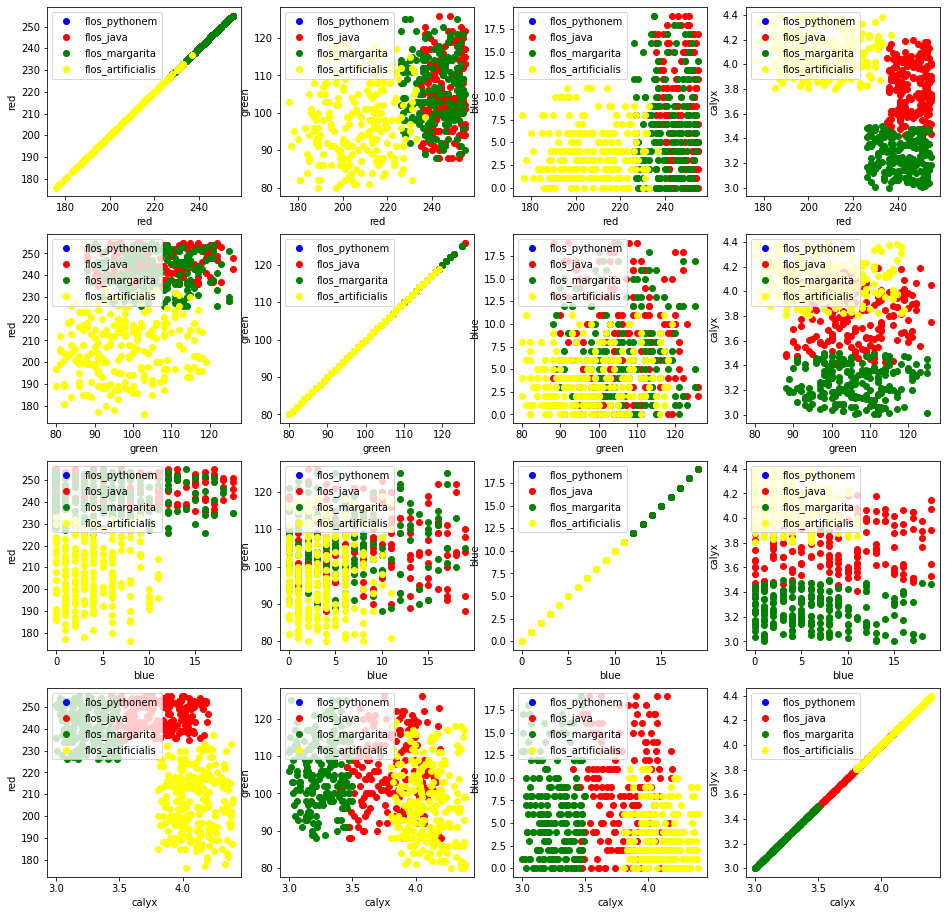### Generate Synthetic Data with Scikit-Learn

It is a lot easier to use the possibilities of Scikit-Learn to create synthetic data.

The functionalities available in sklearn can be grouped into

1. Generators for classifictation and clustering
2. Generators for creating data for regression
3. Generators for manifold learning¶
4. Generators for decomposition

#### Generators for Classification and Clustering

We start with the the function make_blobs of sklearn.datasets to create 'blob' like data distributions. By setting the value of centers to n_classes, we determine the number of blobs, i.e. the clusters. n_samples corresponds to the total number of points equally divided among clusters. If random_state is not set, we will have random results every time we call the function. We pass an int to this parameter for reproducible output across multiple function calls.

import numpy as np
import matplotlib.pyplot as plt
from sklearn.datasets import make_blobs

n_classes = 4
data, labels = make_blobs(n_samples=1000,
centers=n_classes,
random_state=100)

labels[:7]

Output:
array([1, 3, 1, 3, 1, 3, 2])

We will visualize the previously created blob custers with matplotlib:

fig, ax = plt.subplots()

colours = ('green', 'orange', 'blue', "pink")
for label in range(n_classes):
ax.scatter(x=data[labels==label, 0],
y=data[labels==label, 1],
c=colours[label],
s=40,
label=label)

ax.set(xlabel='X',
ylabel='Y',
title='Blobs Examples')

ax.legend(loc='upper right')

Output:
<matplotlib.legend.Legend at 0x7f50f92a4640>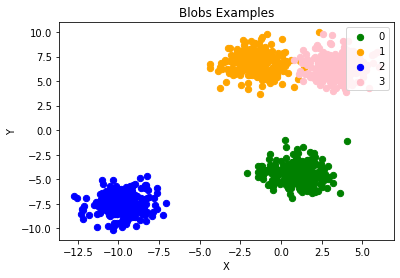The centers of the blobs were randomly chosen in the previous example. In the following example we set the centers of the blobs explicitly. We create a list with the center points and pass it to the parameter centers:

import numpy as np
import matplotlib.pyplot as plt
from sklearn.datasets import make_blobs

centers = [[2, 3], [4, 5], [7, 9]]
data, labels = make_blobs(n_samples=1000,
centers=np.array(centers),
random_state=1)

labels[:7]

Output:
array([0, 1, 1, 0, 2, 2, 2])

Let us have a look at the previously created blob clusters:

fig, ax = plt.subplots()

colours = ('green', 'orange', 'blue')
for label in range(len(centers)):
ax.scatter(x=data[labels==label, 0],
y=data[labels==label, 1],
c=colours[label],
s=40,
label=label)

ax.set(xlabel='X',
ylabel='Y',
title='Blobs Examples')

ax.legend(loc='upper right')

Output:
<matplotlib.legend.Legend at 0x7f50f91eaca0>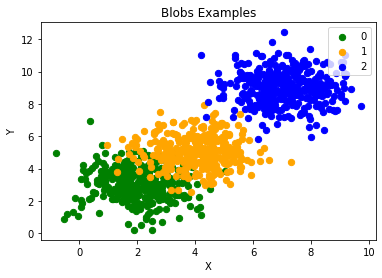Usually, you want to save your artificially created datasets in a file. For this purpose, we can use the function savetxt from numpy. Before we can do this we have to reaarange our data. Each row should contain both the data and the label:

import numpy as np

labels = labels.reshape((labels.shape,1))
all_data = np.concatenate((data, labels), axis=1)
all_data[:7]

Output:
array([[ 1.72415394,  4.22895559,  0.        ],
[ 4.16466507,  5.77817418,  1.        ],
[ 4.51441156,  4.98274913,  1.        ],
[ 1.49102772,  2.83351405,  0.        ],
[ 6.0386362 ,  7.57298437,  2.        ],
[ 5.61044976,  9.83428321,  2.        ],
[ 5.69202866, 10.47239631,  2.        ]])

For some people it might be complicated to understand the combination of reshape and concatenate. Therefore, you can see an extremely simple example in the following code:

import numpy as np

a = np.array( [[1, 2], [3, 4]])
b = np.array( [5, 6])
b = b.reshape((b.shape, 1))
print(b)

x = np.concatenate( (a, b), axis=1)
x

[
]

Output:
array([[1, 2, 5],
[3, 4, 6]])

We use the numpy function savetxt to save the data. Don't worry about the strange name, it is just for fun and for reasons which will be clear soon:

np.savetxt("squirrels.txt",
all_data,
fmt=['%.3f', '%.3f', '%1d'])
all_data[:10]

Output:
array([[ 1.72415394,  4.22895559,  0.        ],
[ 4.16466507,  5.77817418,  1.        ],
[ 4.51441156,  4.98274913,  1.        ],
[ 1.49102772,  2.83351405,  0.        ],
[ 6.0386362 ,  7.57298437,  2.        ],
[ 5.61044976,  9.83428321,  2.        ],
[ 5.69202866, 10.47239631,  2.        ],
[ 6.14017298,  8.56209179,  2.        ],
[ 2.97620068,  5.56776474,  1.        ],
[ 8.27980017,  8.54824406,  2.        ]])

## Reading the data and conversion back into 'data' and 'labels'

We will demonstrate now, how to read in the data again and how to split it into data and labels again:

file_data = np.loadtxt("squirrels.txt")

data = file_data[:,:-1]
labels = file_data[:,2:]

labels = labels.reshape((labels.shape))


We had called the data file squirrels.txt, because we imagined a strange kind of animal living in the Sahara desert. The x-values stand for the night vision capabilities of the animals and the y-values correspond to the colour of the fur, going from sandish to black. We have three kinds of squirrels, 0, 1, and 2. (Be aware that our squirrals are imaginary squirrels and have nothing to do with the real squirrels of the Sahara!)

import matplotlib.pyplot as plt

colours = ('green', 'red', 'blue', 'magenta', 'yellow', 'cyan')
n_classes = 3

fig, ax = plt.subplots()
for n_class in range(0, n_classes):
ax.scatter(data[labels==n_class, 0], data[labels==n_class, 1],
c=colours[n_class], s=10, label=str(n_class))

ax.set(xlabel='Night Vision',
ylabel='Fur color from sandish to black, 0 to 10 ',
title='Sahara Virtual Squirrel')

ax.legend(loc='upper right')

Output:
<matplotlib.legend.Legend at 0x7f545b4d6340>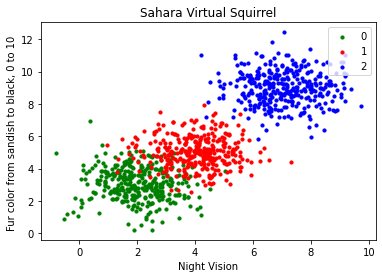We will train our articifical data in the following code:

from sklearn.model_selection import train_test_split

data_sets = train_test_split(data,
labels,
train_size=0.8,
test_size=0.2,
random_state=42 # garantees same output for every run
)

train_data, test_data, train_labels, test_labels = data_sets

# import model
from sklearn.neighbors import KNeighborsClassifier

# create classifier
knn = KNeighborsClassifier(n_neighbors=8)

# train
knn.fit(train_data, train_labels)

# test on test data:
calculated_labels = knn.predict(test_data)
calculated_labels

Output:
array([2., 0., 1., 1., 0., 1., 2., 2., 2., 2., 0., 1., 0., 0., 1., 0., 1.,
2., 0., 0., 1., 2., 1., 2., 2., 1., 2., 0., 0., 2., 0., 2., 2., 0.,
0., 2., 0., 0., 0., 1., 0., 1., 1., 2., 0., 2., 1., 2., 1., 0., 2.,
1., 1., 0., 1., 2., 1., 0., 0., 2., 1., 0., 1., 1., 0., 0., 0., 0.,
0., 0., 0., 1., 1., 0., 1., 1., 1., 0., 1., 2., 1., 2., 0., 2., 1.,
1., 0., 2., 2., 2., 0., 1., 1., 1., 2., 2., 0., 2., 2., 2., 2., 0.,
0., 1., 1., 1., 2., 1., 1., 1., 0., 2., 1., 2., 0., 0., 1., 0., 1.,
0., 2., 2., 2., 1., 1., 1., 0., 2., 1., 2., 2., 1., 2., 0., 2., 0.,
0., 1., 0., 2., 2., 0., 0., 1., 2., 1., 2., 0., 0., 2., 2., 0., 0.,
1., 2., 1., 2., 0., 0., 1., 2., 1., 0., 2., 2., 0., 2., 0., 0., 2.,
1., 0., 0., 0., 0., 2., 2., 1., 0., 2., 2., 1., 2., 0., 1., 1., 1.,
0., 1., 0., 1., 1., 2., 0., 2., 2., 1., 1., 1., 2.])
from sklearn import metrics

print("Accuracy:", metrics.accuracy_score(test_labels, calculated_labels))

Accuracy: 0.97


## Other Interesting Distributions

import numpy as np

import sklearn.datasets as ds
data, labels = ds.make_moons(n_samples=150,
shuffle=True,
noise=0.19,
random_state=None)

data += np.array(-np.ndarray.min(data[:,0]),
-np.ndarray.min(data[:,1]))

np.ndarray.min(data[:,0]), np.ndarray.min(data[:,1])

Output:
(0.0, 0.34649342272719386)
import matplotlib.pyplot as plt
fig, ax = plt.subplots()

ax.scatter(data[labels==0, 0], data[labels==0, 1],
c='orange', s=40, label='oranges')
ax.scatter(data[labels==1, 0], data[labels==1, 1],
c='blue', s=40, label='blues')

ax.set(xlabel='X',
ylabel='Y',
title='Moons')

#ax.legend(loc='upper right');

Output:
[Text(0.5, 0, 'X'), Text(0, 0.5, 'Y'), Text(0.5, 1.0, 'Moons')]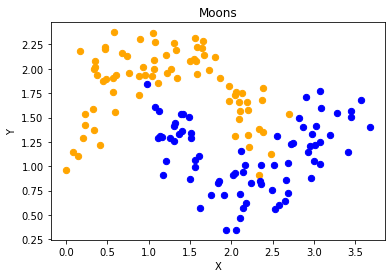We want to scale values that are in a range [min, max] in a range [a, b].

$$f(x) = \frac{(b-a)\cdot(x - min)}{max - min} + a$$

We now use this formula to transform both the X and Y coordinates of data into other ranges:

min_x_new, max_x_new = 33, 88
min_y_new, max_y_new = 12, 20

data, labels = ds.make_moons(n_samples=100,
shuffle=True,
noise=0.05,
random_state=None)

min_x, min_y = np.ndarray.min(data[:,0]), np.ndarray.min(data[:,1])
max_x, max_y = np.ndarray.max(data[:,0]), np.ndarray.max(data[:,1])

#data -= np.array([min_x, 0])
#data *= np.array([(max_x_new - min_x_new) / (max_x - min_x), 1])
#data += np.array([min_x_new, 0])

#data -= np.array([0, min_y])
#data *= np.array([1, (max_y_new - min_y_new) / (max_y - min_y)])
#data += np.array([0, min_y_new])

data -= np.array([min_x, min_y])
data *= np.array([(max_x_new - min_x_new) / (max_x - min_x), (max_y_new - min_y_new) / (max_y - min_y)])
data += np.array([min_x_new, min_y_new])

#np.ndarray.min(data[:,0]), np.ndarray.max(data[:,0])
data[:6]

Output:
array([[71.14479608, 12.28919998],
[62.16584307, 18.75442981],
[61.02613211, 12.80794358],
[64.30752046, 12.32563839],
[81.41469127, 13.64613406],
[82.03929032, 13.63156545]])
def scale_data(data, new_limits, inplace=False ):
if not inplace:
data = data.copy()
min_x, min_y = np.ndarray.min(data[:,0]), np.ndarray.min(data[:,1])
max_x, max_y = np.ndarray.max(data[:,0]), np.ndarray.max(data[:,1])
min_x_new, max_x_new = new_limits
min_y_new, max_y_new = new_limits
data -= np.array([min_x, min_y])
data *= np.array([(max_x_new - min_x_new) / (max_x - min_x), (max_y_new - min_y_new) / (max_y - min_y)])
data += np.array([min_x_new, min_y_new])
if inplace:
return None
else:
return data

data, labels = ds.make_moons(n_samples=100,
shuffle=True,
noise=0.05,
random_state=None)

scale_data(data, [(1, 4), (3, 8)], inplace=True)
data[:10]

Output:
array([[1.19312571, 6.70797983],
[2.74306138, 6.74830445],
[1.15255757, 6.31893824],
[1.03927303, 4.83714182],
[2.91313352, 6.44139267],
[2.13227292, 5.120716  ],
[2.65590196, 3.49417953],
[2.98349928, 5.02232383],
[3.35660593, 3.34679462],
[2.15813861, 4.8036458 ]])
fig, ax = plt.subplots()

ax.scatter(data[labels==0, 0], data[labels==0, 1],
c='orange', s=40, label='oranges')
ax.scatter(data[labels==1, 0], data[labels==1, 1],
c='blue', s=40, label='blues')

ax.set(xlabel='X',
ylabel='Y',
title='moons')

ax.legend(loc='upper right');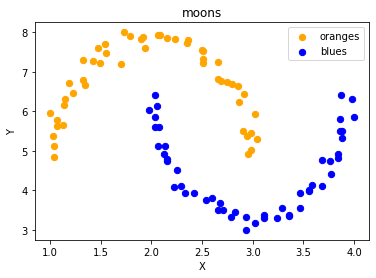import sklearn.datasets as ds
data, labels = ds.make_circles(n_samples=100,
shuffle=True,
noise=0.05,
random_state=None)

fig, ax = plt.subplots()

ax.scatter(data[labels==0, 0], data[labels==0, 1],
c='orange', s=40, label='oranges')
ax.scatter(data[labels==1, 0], data[labels==1, 1],
c='blue', s=40, label='blues')

ax.set(xlabel='X',
ylabel='Y',
title='circles')

ax.legend(loc='upper right')

Output:
<matplotlib.legend.Legend at 0x7f54588c2e20>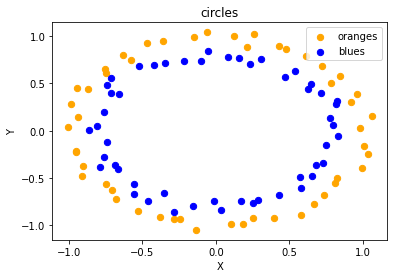print(__doc__)

import matplotlib.pyplot as plt

from sklearn.datasets import make_classification
from sklearn.datasets import make_blobs
from sklearn.datasets import make_gaussian_quantiles

plt.figure(figsize=(8, 8))

plt.subplot(321)
plt.title("One informative feature, one cluster per class", fontsize='small')
X1, Y1 = make_classification(n_features=2, n_redundant=0, n_informative=1,
n_clusters_per_class=1)
plt.scatter(X1[:, 0], X1[:, 1], marker='o', c=Y1,
s=25, edgecolor='k')

plt.subplot(322)
plt.title("Two informative features, one cluster per class", fontsize='small')
X1, Y1 = make_classification(n_features=2, n_redundant=0, n_informative=2,
n_clusters_per_class=1)
plt.scatter(X1[:, 0], X1[:, 1], marker='o', c=Y1,
s=25, edgecolor='k')

plt.subplot(323)
plt.title("Two informative features, two clusters per class",
fontsize='small')
X2, Y2 = make_classification(n_features=2,
n_redundant=0,
n_informative=2)
plt.scatter(X2[:, 0], X2[:, 1], marker='o', c=Y2,
s=25, edgecolor='k')

plt.subplot(324)
plt.title("Multi-class, two informative features, one cluster",
fontsize='small')
X1, Y1 = make_classification(n_features=2,
n_redundant=0,
n_informative=2,
n_clusters_per_class=1,
n_classes=3)
plt.scatter(X1[:, 0], X1[:, 1], marker='o', c=Y1,
s=25, edgecolor='k')

plt.subplot(325)
plt.title("Gaussian divided into three quantiles", fontsize='small')
X1, Y1 = make_gaussian_quantiles(n_features=2, n_classes=3)
plt.scatter(X1[:, 0], X1[:, 1], marker='o', c=Y1,
s=25, edgecolor='k')

plt.show()

Automatically created module for IPython interactive environment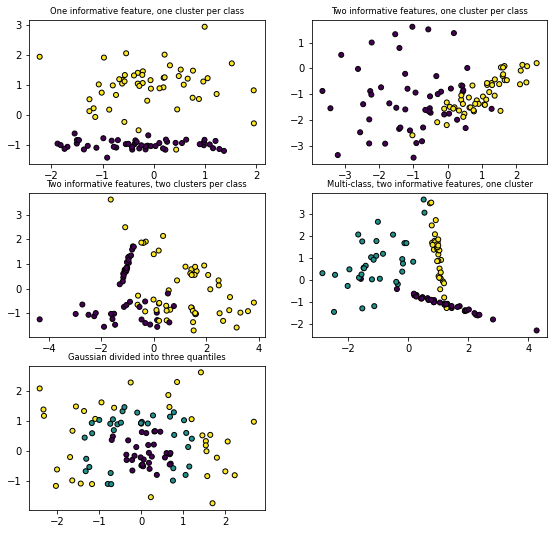### Exercises

#### Exercise 1

Create two testsets which are separable with a perceptron without a bias node.

#### Exercise 2

Create two testsets which are not separable with a dividing line going through the origin.

#### Exercise 3

Create a dataset with five classes "Tiger", "Lion", "Penguin", "Dolphin", and "Python". The sets should look similar to the following diagram:### Solutions

#### Solution to Exercise 1

data, labels = make_blobs(n_samples=100,
cluster_std = 0.5,
centers=[[1, 4] ,[4, 1]],
random_state=1)

fig, ax = plt.subplots()

colours = ["orange", "green"]
label_name = ["Tigers", "Lions"]
for label in range(0, 2):
ax.scatter(data[labels==label, 0], data[labels==label, 1],
c=colours[label], s=40, label=label_name[label])

ax.set(xlabel='X',
ylabel='Y',
title='dataset')

ax.legend(loc='upper right')

Output:
<matplotlib.legend.Legend at 0x7f788afb2c40>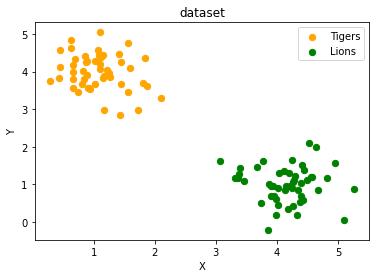#### Solution to Exercise 2

data, labels = make_blobs(n_samples=100,
cluster_std = 0.5,
centers=[[2, 2] ,[4, 4]],
random_state=1)

fig, ax = plt.subplots()

colours = ["orange", "green"]
label_name = ["label0", "label1"]
for label in range(0, 2):
ax.scatter(data[labels==label, 0], data[labels==label, 1],
c=colours[label], s=40, label=label_name[label])

ax.set(xlabel='X',
ylabel='Y',
title='dataset')

ax.legend(loc='upper right')

Output:
<matplotlib.legend.Legend at 0x7f788af8eac0>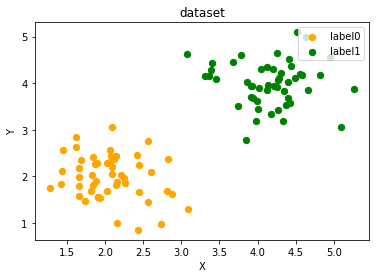#### Solution to Exercise 3

import sklearn.datasets as ds
data, labels = ds.make_circles(n_samples=100,
shuffle=True,
noise=0.05,
random_state=42)

centers = [[3, 4], [5, 3], [4.5, 6]]
data2, labels2 = make_blobs(n_samples=100,
cluster_std = 0.5,
centers=centers,
random_state=1)

for i in range(len(centers)-1, -1, -1):
labels2[labels2==0+i] = i+2

print(labels2)
labels = np.concatenate([labels, labels2])
data = data * [1.2, 1.8] + [3, 4]

data = np.concatenate([data, data2], axis=0)

[2 4 4 3 4 4 3 3 2 4 4 2 4 4 3 4 2 4 4 4 4 2 2 4 4 3 2 2 3 2 2 3 2 3 3 3 3
3 4 3 3 2 3 3 3 2 2 2 2 3 4 4 4 2 4 3 3 2 2 3 4 4 3 3 4 2 4 2 4 3 3 4 2 2
3 4 4 2 3 2 3 3 4 2 2 2 2 3 2 4 2 2 3 3 4 4 2 2 4 3]

fig, ax = plt.subplots()

colours = ["orange", "blue", "magenta", "yellow", "green"]
label_name = ["Tiger", "Lion", "Penguin", "Dolphin", "Python"]
for label in range(0, len(centers)+2):
ax.scatter(data[labels==label, 0], data[labels==label, 1],
c=colours[label], s=40, label=label_name[label])

ax.set(xlabel='X',
ylabel='Y',
title='dataset')

ax.legend(loc='upper right')

Output:
<matplotlib.legend.Legend at 0x7f788b1d42b0>